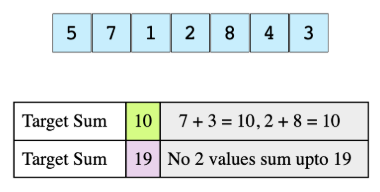# Sum of Two Values

## Problem Statement

Given an array of integers and a value, determine if there are any two integers in the array whose sum is equal to the given value. Return true if the sum exists and return false if it does not.

Consider this array and the target sums:## Hints

• Use hashing
• Use comparison between elements

## Try it yourself

bool find_sum_of_two(vector<int>& A, int val) {  //TODO: Write - Your - Code  return false;}

## Solution

bool find_sum_of_two(vector<int>& A, int val) {  unordered_set<int> found_values;  for (int& a : A) {    if (found_values.find(val - a) != found_values.end()) {      return true;    }    found_values.insert(a);  }  return false;}int main() {  vector<int> v = {5, 7, 1, 2, 8, 4, 3};  vector<int> test = {3, 20, 1, 2, 7};  for(int i=0; i<test.size(); i++){    bool output = find_sum_of_two(v, test[i]);    cout << "find_sum_of_two(v, " << test[i] << ") = " << (output ? "true" : "false") << endl;  }  return 0;}

## Solution Explanation

### Runtime Complexity

The runtime complexity of this solution is linear, O(n).

### Memory Complexity

The memory complexity of this solution is linear, O(n).

### Solution Breakdown

In this solution, you can use the following algorithm to find a pair that add up to the target (say val).

• Scan the whole array once and store visited elements in a hash set.
• During scan, for every element e in the array, we check if val - e is present in the hash set i.e. val - e is already visited.
• If val - e is found in the hash set, it means there is a pair (e, val - e) in array whose sum is equal to the given val.
• If we have exhausted all elements in the array and didn’t find any such pair, the function will return false.

Practice problems like this and many more by checking out our Grokking the Coding Interview: Patterns for Coding Questions course!

Learn in-demand tech skills in half the time